Smartick is an advanced online program that teaches kids math and coding in only 15 min. a day
Try it for free!

Dec15

# Practice 3-Digit Division Examples

In this post, we’re going to see another example of 3-digit division that’s a little more complicated.

### We’re going to go ahead and do a 3-digit division problem step by step.

Step 1. Count how many digits the divisor has.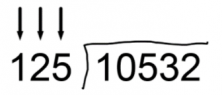Step 2. Use the same number of digits the dividend has for the divisor. In this example, we’ll use 3 digits.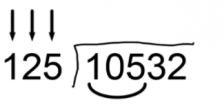Step 3. Compare the dividend’s digits with the divisor’s.

• If the dividend’s number is bigger than the divisor’s (in our example, the dividend is 105), we can start dividing.
• If the dividend’s number is smaller than the divisor’s (the divisor is 125), we need to take another digit from the dividend to have a bigger number. In this example, 105 is smaller than 125, so we need to take another number. Now, 125 fits into 1053 with no problem.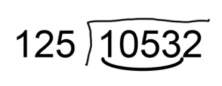Step 4. Divide the dividend’s numbers by the divisor’s.

To know which number to put in the quotient, we need to divide the first number of the dividend, in this example, we’ll need to take the first two numbers because we had to take 4 digits from the dividend.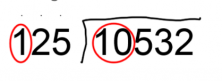So, we divide 10 by 1 and it gives us 10. However, if we multiply 125 x 10, it gives us 1250 and it doesn’t fit in the 4 numbers anymore (1053). So, we need to use a smaller number, 9. We go ahead and multiply 125 x 9 = 1125. Once again, 1125 doesn’t fit into 1053. We must try again with a smaller number, 8.

125 x 8 = 1000. This one works because it’s less than 1053!

We need to write the 8 in the quotient and the result from 125 x 8 underneath the dividend. Now, we just subtract.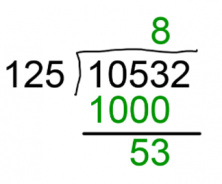Step 5. Bring down the dividend’s next digit and repeat the previous step until there aren’t any more numbers to bring down.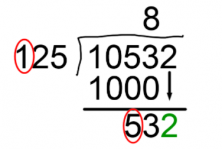We need to bring down the 2. Now, if we divide 5 by 1, we get 5. We go on to multiply 125 x 5 and see if it fits into 532.

125 x 5 = 625.

625 is greater than 532. So, let’s try a smaller number, 4.

125 x 4 = 500.

500 fits into 532 with no problem. Now, we write the 4 in the quotient.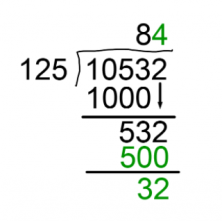Now, we’re done dividing 10532 by 125 because there aren’t any more digits left in the dividend to bring down and 125 can’t fit into 32. The answer is 84 with a remainder of 32.

And now, as always, we’re going to check our answer to see if we divided everything correctly.

divisor x quotient + remainder = dividend

125 x 84 + 32 = 10532

The check is a success!

If you want to keep learning 3-Digit Division and lots of elementary math, make an account at Smartick and try it for free!

Learn More:

Latest posts by Smartick (see all)

2 Comments

• RoseSep 24 2019, 1:17 PM

Thanks for the useful content

Reply
• sribash senOct 07 2018, 9:24 AM

excellent

Reply